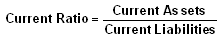# Current Ratio

Current Ratio
A liquidity ratio that measures a company's ability to pay short-term obligations.

The Current Ratio formula is:Also known as "liquidity ratio", "cash asset ratio" and "cash ratio".

The ratio is mainly used to give an idea of the company's ability to pay back its short-term liabilities (debt and payables) with its short-term assets (cash, inventory, receivables). The higher the current ratio, the more capable the company is of paying its obligations. A ratio under 1 suggests that the company would be unable to pay off its obligations if they came due at that point. While this shows the company is not in good financial health, it does not necessarily mean that it will go bankrupt - as there are many ways to access financing - but it is definitely not a good sign.

The current ratio can give a sense of the efficiency of a company's operating cycle or its ability to turn its product into cash. Companies that have trouble getting paid on their receivables or have long inventory turnover can run into liquidity problems because they are unable to alleviate their obligations. Because business operations differ in each industry, it is always more useful to compare companies within the same industry.

This ratio is similar to the acid-test ratio except that the acid-test ratio does not include inventory and prepaids as assets that can be liquidated. The components of current ratio (current assets and current liabilities) can be used to derive working capital (difference between current assets and current liabilities). Working capital is frequently used to derive the working capital ratio, which is working capital as a ratio of sales.

Investment dictionary. . 2012.

### Look at other dictionaries:

• current ratio — The ratio obtained by dividing current assets by current liabilities to measure ability of the firm to pay short term debt from readily available funds. Related links gearing Practical Law Dictionary. Glossary of UK, US and international legal… …   Law dictionary

• current ratio — The ratio obtained when total current assets are divided by total current liabilities. A commonly used but not always good proxy for a firm s liquidity. American Banker Glossary Indicator of short term debt paying ability. Determined by dividing… …   Financial and business terms

• Current ratio — The current ratio is a financial ratio that measures whether or not a firm has enough resources to pay its debts over the next 12 months. It compares a firm s current assets to its current liabilities. It is expressed as follows: For example, if… …   Wikipedia

• Current ratio — Indicator of short term debt paying ability. Determined by dividing current assets by current liabilities. The higher the ratio, the more liquid the company. The New York Times Financial Glossary * * * current ratio current ratio ➔ ratio * * *… …   Financial and business terms

• Current Ratio — Liquiditätsgrade sind insbesondere in der Unternehmensfinanzierung und der Finanzbuchhaltung verwendete betriebswirtschaftliche Kennzahlen, mit denen die Fähigkeit eines Unternehmens, seinen Zahlungsverpflichtungen fristgerecht nachzukommen,… …   Deutsch Wikipedia

• current ratio — working capital ratio The ratio of the current assets of a business to the current liabilities, expressed as x:1 and used as a test of liquidity. For example, if the current assets are £250,000 and the current liabilities are £125,000 the current …   Accounting dictionary

• current ratio — working capital ratio The ratio of the current assets of a business to the current liabilities, expressed as x:1 and used as a test of liquidity. For example, if the current assets are £250, 000 and the current liabilities are £125, 000 the… …   Big dictionary of business and management

• current ratio — noun : the ratio between current assets and liabilities used in appraising credit worthiness of a business * * * the ratio between current assets and current liabilities. * * * current ratio, the ratio of current assets to current liabilities …   Useful english dictionary

• Current ratio —   The ratio of current assets divided by current liabilities that shows the ability of a utility to pay its current obligations from its current assets. A measure of liquidity, the higher the ratio, the more assurance that current liabilities can …   Energy terms

• current ratio — the ratio between current assets and current liabilities. * * * …   Universalium